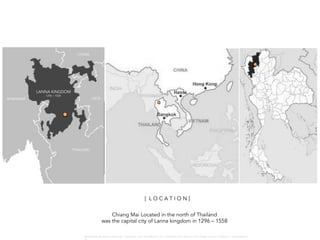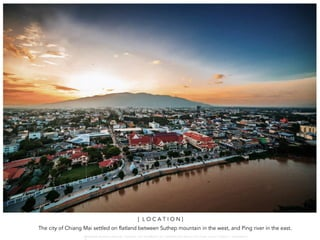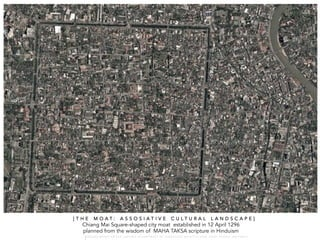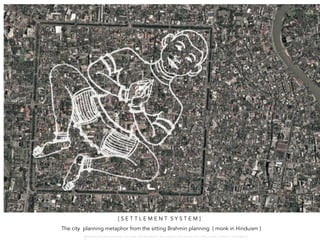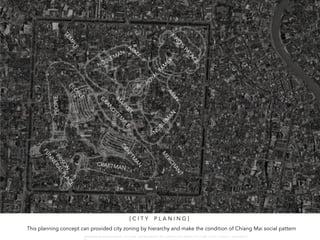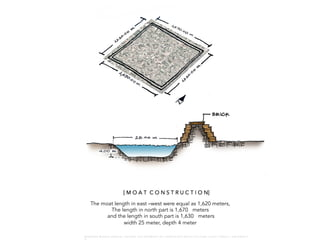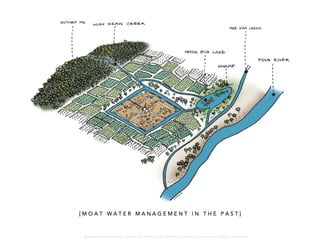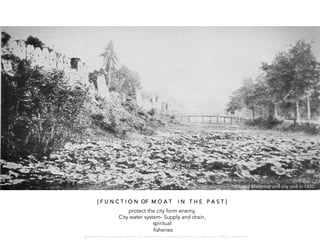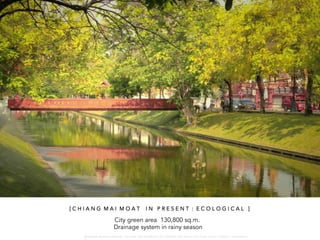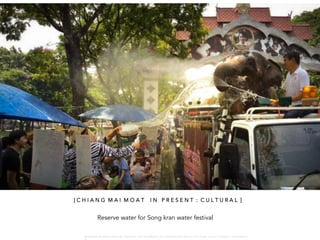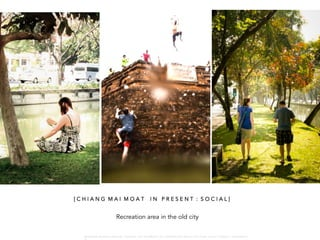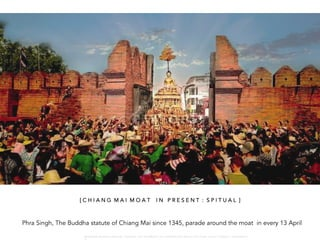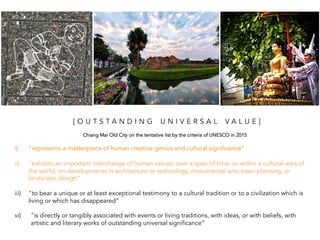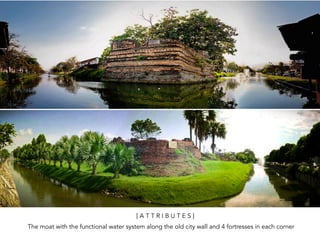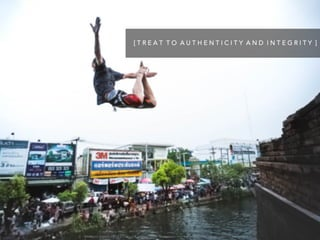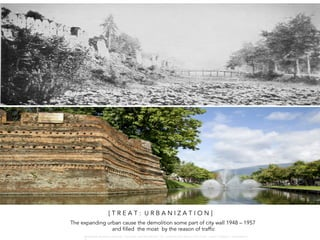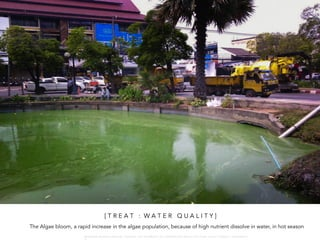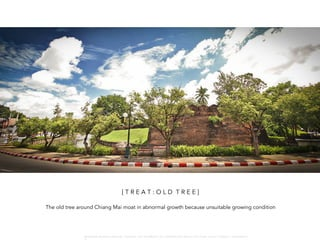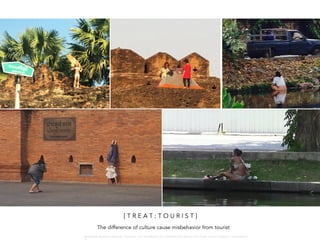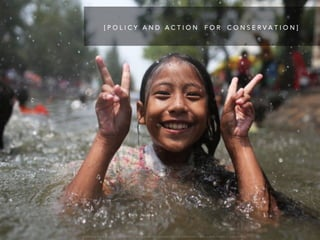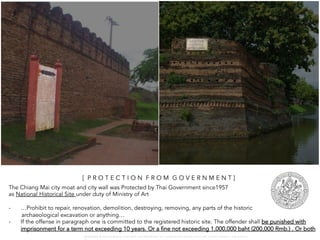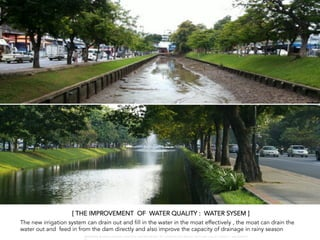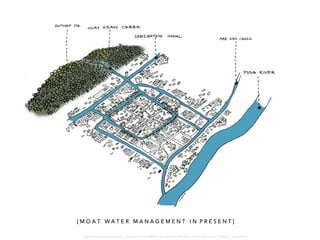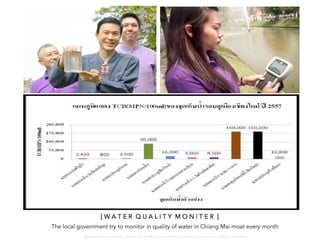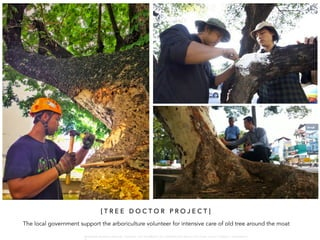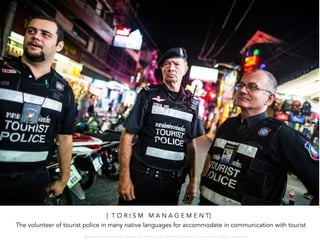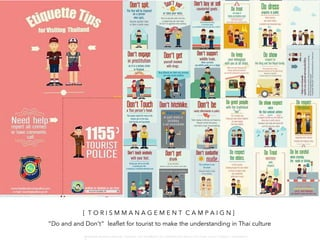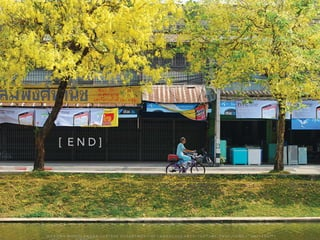1 de 29

### CM moat Cul Lan .pdf

1. C H I A N G M A I C I T Y M O A T T H E C U L T U R A L L A N D S C A S C A P E O F W A R O N G W O N G L A N G K A : 1 6 9 1 0 0 4 D E P A R T M E N T O F L A N D S C A P E A R C H I T E C T U R E , C A U P , T O N G J I U N I V E R S I T Y
2. Chiang Mai Located in the north of Thailand was the capital city of Lanna kingdom in 1296 – 1558 LANNA KINGDOM MYANMAR THAILAND LAOS CHINA 1296 – 1558 [ L O C A T I O N ] W A R O N G W O N G L A N G K A : 1 6 9 1 0 0 4 D E P A R T M E N T O F L A N D S C A P E A R C H I T E C T U R E , C A U P , T O N G J I U N I V E R S I T
3. The city of Chiang Mai settled on flatland between Suthep mountain in the west, and Ping river in the east. [ L O C A T I O N ] W A R O N G W O N G L A N G K A : 1 6 9 1 0 0 4 D E P A R T M E N T O F L A N D S C A P E A R C H I T E C T U R E , C A U P , T O N G J I U N I V E R S I T
4. Chiang Mai Square-shaped city moat established in 12 April 1296 planned from the wisdom of MAHA TAKSA scripture in Hinduism [ T H E M O A T : A S S O S I A T I V E C U L T U R A L L A N D S C A P E ] W A R O N G W O N G L A N G K A : 1 6 9 1 0 0 4 D E P A R T M E N T O F L A N D S C A P E A R C H I T E C T U R E , C A U P , T O N G J I U N I V E R S I T
5. [ S E T T L E M E N T S Y S T E M ] The city planning metaphor from the sitting Brahmin planning ( monk in Hinduism ) W A R O N G W O N G L A N G K A : 1 6 9 1 0 0 4 D E P A R T M E N T O F L A N D S C A P E A R C H I T E C T U R E , C A U P , T O N G J I U N I V E R S I T
6. CRAFTMAN PE O PL E [ C I T Y P L A N I N G ] This planning concept can provided city zoning by hierarchy and make the condition of Chiang Mai social pattern W A R O N G W O N G L A N G K A : 1 6 9 1 0 0 4 D E P A R T M E N T O F L A N D S C A P E A R C H I T E C T U R E , C A U P , T O N G J I U N I V E R S I T
7. [ M O A T C O N S T R U C T I O N] The moat length in east –west were equal as 1,620 meters, The length in north part is 1,670 meters and the length in south part is 1,630 meters width 25 meter, depth 4 meter W A R O N G W O N G L A N G K A : 1 6 9 1 0 0 4 D E P A R T M E N T O F L A N D S C A P E A R C H I T E C T U R E , C A U P , T O N G J I U N I V E R S I T
8. [ M O A T W A T E R M A N A G E M E N T I N T H E P A S T ] W A R O N G W O N G L A N G K A : 1 6 9 1 0 0 4 D E P A R T M E N T O F L A N D S C A P E A R C H I T E C T U R E , C A U P , T O N G J I U N I V E R S I T
9. [ F U N C T I O N OF M O A T I N T H E P A S T ] protect the city form enemy, City water system- Supply and drain, spiritual fisheries Chiang Mai moat and city wall in 1850 W A R O N G W O N G L A N G K A : 1 6 9 1 0 0 4 D E P A R T M E N T O F L A N D S C A P E A R C H I T E C T U R E , C A U P , T O N G J I U N I V E R S I T
10. [ C H I A N G M A I M O A T I N P R E S E N T : E C O L O G I C A L ] City green area 130,800 sq.m. Drainage system in rainy season W A R O N G W O N G L A N G K A : 1 6 9 1 0 0 4 D E P A R T M E N T O F L A N D S C A P E A R C H I T E C T U R E , C A U P , T O N G J I U N I V E R S I T
11. Reserve water for Song kran water festival [ C H I A N G M A I M O A T I N P R E S E N T : C U L T U R A L ] W A R O N G W O N G L A N G K A : 1 6 9 1 0 0 4 D E P A R T M E N T O F L A N D S C A P E A R C H I T E C T U R E , C A U P , T O N G J I U N I V E R S I T
12. Recreation area in the old city [ C H I A N G M A I M O A T I N P R E S E N T : S O C I A L ] W A R O N G W O N G L A N G K A : 1 6 9 1 0 0 4 D E P A R T M E N T O F L A N D S C A P E A R C H I T E C T U R E , C A U P , T O N G J I U N I V E R S I T
13. Phra Singh, The Buddha statute of Chiang Mai since 1345, parade around the moat in every 13 April [ C H I A N G M A I M O A T I N P R E S E N T : S P I T U A L ] W A R O N G W O N G L A N G K A : 1 6 9 1 0 0 4 D E P A R T M E N T O F L A N D S C A P E A R C H I T E C T U R E , C A U P , T O N G J I U N I V E R S I T
14. i) "represents a masterpiece of human creative genius and cultural significance” ii) "exhibits an important interchange of human values, over a span of time, or within a cultural area of the world, on developments in architecture or technology, monumental arts, town-planning, or landscape design” iii) "to bear a unique or at least exceptional testimony to a cultural tradition or to a civilization which is living or which has disappeared” vi) "is directly or tangibly associated with events or living traditions, with ideas, or with beliefs, with artistic and literary works of outstanding universal significance” [ O U T S T A N D I N G U N I V E R S A L V A L U E ] Chiang Mai Old City on the tentative list by the criteria of UNESCO in 2015
15. [ A T T R I B U T E S ] The moat with the functional water system along the old city wall and 4 fortresses in each corner
16. [ T R E A T T O A U T H E N T I C I T Y A N D I N T E G R I T Y ] W A R O N G W O N G L A N G K A : 1 6 9 1 0 0 4 D E P A R T M E N T O F L A N D S C A P E A R C H I T E C T U R E , C A U P , T O N G J I U N I V E R S I T
17. [ T R E A T : U R B A N I Z A T I O N ] The expanding urban cause the demolition some part of city wall 1948 – 1957 and filled the moat by the reason of traffic W A R O N G W O N G L A N G K A : 1 6 9 1 0 0 4 D E P A R T M E N T O F L A N D S C A P E A R C H I T E C T U R E , C A U P , T O N G J I U N I V E R S I T
18. [ T R E A T : W A T E R Q U A L I T Y ] The Algae bloom, a rapid increase in the algae population, because of high nutrient dissolve in water, in hot season W A R O N G W O N G L A N G K A : 1 6 9 1 0 0 4 D E P A R T M E N T O F L A N D S C A P E A R C H I T E C T U R E , C A U P , T O N G J I U N I V E R S I T
19. [ T R E A T : O L D T R E E ] The old tree around Chiang Mai moat in abnormal growth because unsuitable growing condition W A R O N G W O N G L A N G K A : 1 6 9 1 0 0 4 D E P A R T M E N T O F L A N D S C A P E A R C H I T E C T U R E , C A U P , T O N G J I U N I V E R S I T
20. [ T R E A T : T O U R I S T ] The difference of culture cause misbehavior from tourist W A R O N G W O N G L A N G K A : 1 6 9 1 0 0 4 D E P A R T M E N T O F L A N D S C A P E A R C H I T E C T U R E , C A U P , T O N G J I U N I V E R S I T
21. [ P O L I C Y A N D A C T I O N F O R C O N S E R V A T I O N ] W A R O N G W O N G L A N G K A : 1 6 9 1 0 0 4 D E P A R T M E N T O F L A N D S C A P E A R C H I T E C T U R E , C A U P , T O N G J I U N I V E R S I T
22. The Chiang Mai city moat and city wall was Protected by Thai Government since1957 as National Historical Site under duty of Ministry of Art - …Prohibit to repair, renovation, demolition, destroying, removing, any parts of the historic archaeological excavation or anything… - If the offense in paragraph one is committed to the registered historic site. The offender shall be punished with imprisonment for a term not exceeding 10 years. Or a fine not exceeding 1,000,000 baht (200,000 Rmb.) . Or both [ P R O T E C T I O N F R O M G O V E R N M E N T ] W A R O N G W O N G L A N G K A : 1 6 9 1 0 0 4 D E P A R T M E N T O F L A N D S C A P E A R C H I T E C T U R E , C A U P , T O N G J I U N I V E R S I T
23. [ THE IMPROVEMENT OF WATER QUALITY : WATER SYSEM ] The new irrigation system can drain out and fill in the water in the moat effectively , the moat can drain the water out and feed in from the dam directly and also improve the capacity of drainage in rainy season W A R O N G W O N G L A N G K A : 1 6 9 1 0 0 4 D E P A R T M E N T O F L A N D S C A P E A R C H I T E C T U R E , C A U P , T O N G J I U N I V E R S I T
24. [ M O A T W A T E R M A N A G E M E N T I N P R E S E N T ] W A R O N G W O N G L A N G K A : 1 6 9 1 0 0 4 D E P A R T M E N T O F L A N D S C A P E A R C H I T E C T U R E , C A U P , T O N G J I U N I V E R S I T
25. [ W A T E R Q U A L I T Y M O N I T E R ] The local government try to monitor in quality of water in Chiang Mai moat every month W A R O N G W O N G L A N G K A : 1 6 9 1 0 0 4 D E P A R T M E N T O F L A N D S C A P E A R C H I T E C T U R E , C A U P , T O N G J I U N I V E R S I T
26. [ T R E E D O C T O R P R O J E C T ] The local government support the arboriculture volunteer for intensive care of old tree around the moat W A R O N G W O N G L A N G K A : 1 6 9 1 0 0 4 D E P A R T M E N T O F L A N D S C A P E A R C H I T E C T U R E , C A U P , T O N G J I U N I V E R S I T
27. [ P R O T E C T I O N F R O M G O V E R N M E N T ] [ T O R I S M M A N A G E M E N T] The volunteer of tourist police in many native languages for accommodate in communication with tourist W A R O N G W O N G L A N G K A : 1 6 9 1 0 0 4 D E P A R T M E N T O F L A N D S C A P E A R C H I T E C T U R E , C A U P , T O N G J I U N I V E R S I T
28. [ T O R I S M M A N A G E M E N T C A M P A I G N ] “Do and and Don’t” leaflet for tourist to make the understanding in Thai culture W A R O N G W O N G L A N G K A : 1 6 9 1 0 0 4 D E P A R T M E N T O F L A N D S C A P E A R C H I T E C T U R E , C A U P , T O N G J I U N I V E R S I T
29. [ E N D ] W A R O N G W O N G L A N G K A : 1 6 9 1 0 0 4 D E P A R T M E N T O F L A N D S C A P E A R C H I T E C T U R E , C A U P , T O N G J I U N I V E R S I T Y